Theory and Modern Applications

# Filtering and identification of a state space model with linear and bilinear interactions between the states

## Abstract

In this paper, we introduce a new bilinear model in the state space form. The evolution of this model is linear-bilinear in the state of the system. The classical Kalman filter and smoother are not applicable to this model, and therefore, we derive a new Kalman filter and smoother for our model. The new algorithm depends on a special linearization of the second-order term by making use of the best available information about the state of the system. We also derive the expectation maximization (EM) algorithm for the parameter identification of the model. A Monte Carlo simulation is included to illustrate the efficiency of the proposed algorithm. An application in which we fit a bilinear model to wind speed data taken from actual measurements is included. We compare our model with a linear fit to illustrate the superiority of the bilinear model.

## 1 Introduction

Bilinear systems are a special type of nonlinear systems capable of representing a variety of important physical processes. They are used in many applications in real life such as chemistry, biology, robotics, manufacturing, engineering, and economics  where linear models are ineffective or inadequate. They have also been recently used to analyze and forecast weather conditions .

Bilinear systems have three main advantages over linear ones: Firstly, they describe a wider class of problems of practical importance. Secondly, they provide more flexible approximations to nonlinear systems than linear systems do. Thirdly, one can make use of their rich geometric and algebraic structures, which promises to be a fruitful field of research for scientists  as well as practitioners.

Bilinear models were first introduced in the control theory literature in 1960s . So far, the type of nonlinearity that is extensively treated and analyzed consists of bilinear interaction between the states of the system and the system input [1, 2, 12]. Aside from their practical importance, these systems are easier to handle because they are reducible to linear ones through the use of a certain Kronecker product. In this work, we treat the case where the nonlinearity of the system consists of bilinear interaction between the states of the system themselves. This means that our model will be able to handle evolutions according to the Lotka-Volterra models  or the Lorenz weather models [7, 8, 10], thus enabling a wider and more flexible application of such models. To the best of our knowledge, no attempt has been made to treat such systems in the general setting presented here.

The widespread use of bilinear models motivates the need to develop their parameter identification algorithms. A lot of work exists in the literature which presents methods of estimation and parameter identification of linear and nonlinear systems . The two most widely used techniques fall under the names of least square estimation and maximum likelihood estimation, respectively.

The maximum likelihood estimation is computed through the well-known EM algorithm . It is an iterative method that tries to improve a current estimate of the system parameters by maximizing the underlying likelihood densities. The algorithm is useful in a variety of incomplete data problems, where algorithms such as the Newton-Raphson method may turn out to be more complicated. It consists of two steps called the Expectation step or the E-step and the Maximization step or the M-step; hence the name of the algorithm. This name was first coined by Dempster, Laird, and Rubin in their fundamental paper . In this paper, we develop the EM algorithm for our bilinear system. This will necessitate also the development of a Kalman filter and smoother suitable for the nonlinear system at hand. The direct development of the recursions for the nonlinear filters is very complicated if not impossible altogether. Instead, we develop our recursions based on a linearization of the quadratic term that uses the most current state estimate available.

The remainder of this article is arranged as follows. In Section 2, the bilinear state space model problem is stated along with underlying assumptions. In Section 3, we derive the bilinear Kalman filter and smoother. Section 4 estimates the unknown parameters in the bilinear state space model via the EM algorithm. Section 5 presents a simulation example that produces very satisfactory results. A real world example is given in Section 6.

## 2 The bilinear state space model

In this section, we introduce a bilinear state space model and describe a generalization of the Kalman filter and smoother to this model. Our model subsumes the well-known Lorentz-96 model  for weather forecast, and the Lotka-Volterra evolution equations appear in many applications in chemistry, biology and control [4, 6]. Other types of bilinear models were investigated in [2, 11], where bilinearity occurs because of the interaction between the input and states of the system.

We will adopt the geometric notation as presented in  where the matrix inner product of two random vectors is defined by

$〈x,y〉=E\left(x{y}^{T}\right),$

and

${\parallel x\parallel }^{2}=E\left(x{x}^{T}\right)=〈x,x〉.$

We know that

$〈x,y〉=cov\left(x,y\right)+E\left(x\right)E{\left(y\right)}^{T}.$

Given a sequence $Y=\left\{{y}_{1},{y}_{2},\dots ,{y}_{t}\right\}$ of random vectors, the conditional expectation $E\left(x|Y\right)$ with respect to this inner product is interpreted geometrically as the orthogonal projection of the vector x in the space spanned by the vectors of Y. In particular, if x is uncorrelated with the elements of Y and if it has zero mean, then x is orthogonal to the subspace generated by Y and $E\left(x|Y\right)=0$. We will also use the projection notation

${\pi }_{t}x:={\pi }_{Y}x:=E\left(x|Y\right).$

It is characterized by

$〈x-{\pi }_{t}x,z〉=0,$

for all $z\in \mathcal{M}\left(Y\right)$; the closed subspace of ${L}^{2}$ of all random vectors z which can be written as measurable functions of the elements of Y .

To introduce the model, let us first define the bilinear function $a:{\mathbb{R}}^{n}×{\mathbb{R}}^{n}\to {\mathbb{R}}^{\frac{n\left(n+1\right)}{2}}$ by

$a\left(x,y\right)={\left({x}_{1}{y}_{1},{x}_{1}{y}_{2},\dots ,{x}_{1}{y}_{n},{x}_{2}{y}_{2},{x}_{2}{y}_{3},\dots ,{x}_{2}{y}_{n},\dots ,{x}_{n}{y}_{n}\right)}^{T},$

where $a\left(\cdot ,\cdot \right)$ is similar to the Kronecker product function except that there is no repetition of the entries. Consider the bilinear state space model given by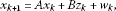(1)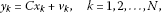(2)

where ${x}_{k}\in {\mathbb{R}}^{n}$ is the state vector, ${y}_{k}\in {\mathbb{R}}^{p}$ is the measurement vector, and ${z}_{k}=a\left({x}_{k},{x}_{k}\right)$ is the bilinear term given by

${z}_{k}=a\left({x}_{k},{x}_{k}\right)={\left({x}_{1}^{2},{x}_{1}{x}_{2},\dots ,{x}_{1}{x}_{n},{x}_{2}^{2},{x}_{2}{x}_{3},\dots ,{x}_{2}{x}_{n},\dots ,{x}_{n}^{2}\right)}^{T}.$

The matrices are of appropriate dimensions, i.e., $A\in {\mathbb{R}}^{n×n}$, $B\in {\mathbb{R}}^{n×\frac{n\left(n+1\right)}{2}}$ and $C\in {\mathbb{R}}^{p×p}$. The uncorrelated noise corruption signals ${w}_{k}$ and ${v}_{k}$ are, as usual, assumed to be white having Gaussian distribution with zero mean and covariances Q and R, respectively, i.e.,

$\begin{array}{c}{w}_{k}\sim N\left(0,Q\right),\phantom{\rule{2em}{0ex}}{v}_{k}\sim N\left(0,R\right),\hfill \\ 〈{w}_{k},{w}_{l}〉=Q{\delta }_{kl},\phantom{\rule{2em}{0ex}}〈{v}_{k},{v}_{l}〉=R{\delta }_{kl}\hfill \end{array}$

and

$〈{w}_{k},{v}_{l}〉=0.$

Lemma 1 $〈{w}_{k},{z}_{l}〉=0$, $〈{v}_{k},{z}_{l}〉=0$, $l\le k$.

Proof Let $l\le k$. Then since ${x}_{l}$, ${w}_{k}$ are uncorrelated and $E\left\{{w}_{k}\right\}=0$, ${w}_{k}\perp {x}_{l}$. This means that $E\left\{{w}_{k}|{x}_{l}\right\}=0$. Hence,

$\begin{array}{rcl}〈{w}_{k},{z}_{l}〉& =& E\left\{E\left\{{w}_{k}a\left({x}_{l},{x}_{l}\right)|{x}_{l}\right\}\right\}\\ =& E\left\{E\left\{{w}_{k}|{x}_{l}\right\}a\left({x}_{l},{x}_{l}\right)\right\}\\ =& 0.\end{array}$

The second equation can be shown in exactly the same way. □

The Taylor polynomial expansion of the form $a\left(x,x\right)$ at the point ${x}_{0}$ can be written as follows (with $z=a\left(x,x\right)$):

$z={z}_{0}+{z}^{\mathrm{\prime }}\left({x}_{0}\right)\left(x-{x}_{0}\right)+\frac{1}{2}\mathcal{H}\left(x,{x}_{0}\right)\left(x-{x}_{0}\right),$
(3)

where ${z}^{\mathrm{\prime }}\left(x\right)$ is the $\frac{n\left(n+1\right)}{2}×n$ gradient of $a\left(x,x\right)$ given by

${z}^{\mathrm{\prime }}\left(x\right)={\left[\frac{\partial {x}_{i}{x}_{j}}{\partial {x}_{l}}\right]}_{i,j,l=1,2,\dots ,m},$

and $\mathcal{H}\left(x,{x}_{0}\right)$ is given by

$\mathcal{H}\left(x,{x}_{0}\right)=\left[\begin{array}{c}{\left(x-{x}_{0}\right)}^{T}{D}_{1}\\ {\left(x-{x}_{0}\right)}^{T}{D}_{2}\\ ⋮\\ {\left(x-{x}_{0}\right)}^{T}{D}_{m}\end{array}\right],$

with ${D}_{k}$ being the matrix of second-order derivatives of the entries of $a\left(x,x\right)$ and $m=\frac{n\left(n+1\right)}{2}$. That is,

To illustrate, suppose $n=3$, then

${z}^{\mathrm{\prime }}\left(x\right)=\left[\begin{array}{ccc}2{x}_{1}& 0& 0\\ {x}_{2}& {x}_{1}& 0\\ {x}_{3}& 0& {x}_{1}\\ 0& 2{x}_{2}& 0\\ 0& {x}_{3}& {x}_{2}\\ 0& 0& 2{x}_{3}\end{array}\right]$

and

$\begin{array}{c}{D}_{1}=\left[\begin{array}{ccc}2& 0& 0\\ 0& 0& 0\\ 0& 0& 0\end{array}\right],\phantom{\rule{2em}{0ex}}{D}_{2}=\left[\begin{array}{ccc}0& 1& 0\\ 1& 0& 0\\ 0& 0& 0\end{array}\right],\phantom{\rule{2em}{0ex}}{D}_{3}=\left[\begin{array}{ccc}0& 0& 1\\ 0& 0& 0\\ 1& 0& 0\end{array}\right],\hfill \\ {D}_{4}=\left[\begin{array}{ccc}0& 0& 0\\ 0& 2& 0\\ 0& 0& 0\end{array}\right],\phantom{\rule{2em}{0ex}}{D}_{5}=\left[\begin{array}{ccc}0& 0& 0\\ 0& 0& 1\\ 0& 1& 0\end{array}\right],\phantom{\rule{2em}{0ex}}{D}_{6}=\left[\begin{array}{ccc}0& 0& 0\\ 0& 0& 0\\ 0& 0& 2\end{array}\right].\hfill \end{array}$

Note, for example, that the Lorentz-96 model (with $n=3$) takes the form (1) with $A=-I$ and

$B=\left[\begin{array}{cccccc}0& 0& 0& 0& 0& 0\\ 0& 0& 0& 0& 0& 0\\ 0& 1& 0& 0& 1& 0\end{array}\right].$

## 3 A bilinear Kalman filter and smoother

In this section, we will develop a Kalman filter and smoother for the bilinear system (1) and (2).

### 3.1 A bilinear Kalman filter

Given a sequence of measurements ${Y}_{t}=\left\{{y}_{1},{y}_{2},\dots ,{y}_{t}\right\}$, let

$\begin{array}{c}{x}_{k}^{t}=E\left({x}_{k}|{Y}_{t}\right):={E}_{t}\left({x}_{k}\right),\hfill \\ {P}_{k}^{t}={\parallel {x}_{k}-{x}_{k}^{t}\parallel }^{2},\hfill \\ {z}_{k}^{t}={E}_{t}\left({z}_{k}\right),\hfill \\ {\stackrel{˙}{P}}_{k}^{t}=〈{x}_{k}-{x}_{k}^{t},{z}_{k}-{z}_{k}^{t}〉,\hfill \\ {\stackrel{¨}{P}}_{k}^{t}={\parallel {z}_{k}-{z}_{k}^{t}\parallel }^{2}.\hfill \end{array}$

When $x={x}_{k}$, equation (3) becomes

$z={z}_{0}+{z}^{\prime }\left({x}_{0}\right)\left({x}_{k}-{x}_{0}\right)+\frac{1}{2}\mathcal{H}\left({x}_{k},{x}_{0}\right)\left({x}_{k}-{x}_{0}\right).$
(4)

In order to compute equation (4), we approximate the second-degree term $\mathcal{H}\left({x}_{k},{x}_{0}\right)$ by using the most current available state estimation for ${x}_{k}$; that is,

• In the case of prediction, we take

By setting ${x}_{0}={x}_{k}^{k-1}$, equation (4) becomes

${z}_{k}\approx {z}_{k}^{k-1}+{z}^{\mathrm{\prime }}\left({x}_{k}^{k-1}\right)\left({x}_{k}-{x}_{k}^{k-1}\right)+\frac{1}{2}\mathcal{H}\left({x}_{k}^{k-2},{x}_{k}^{k-1}\right)\left({x}_{k}-{x}_{k}^{k-1}\right).$
• In the case of filtering, we take

By setting ${x}_{0}={x}_{k}^{k}$, equation (4) becomes

${z}_{k}\approx {z}_{k}^{k}+{z}^{\mathrm{\prime }}\left({x}_{k}^{k}\right)\left({x}_{k}-{x}_{k}^{k}\right)+\frac{1}{2}\mathcal{H}\left({x}_{k}^{k-1},{x}_{k}^{k}\right)\left({x}_{k}-{x}_{k}^{k}\right).$
• In the case of smoothing, we take

By setting ${x}_{0}={x}_{k}^{k+1}$, equation (4) becomes

${z}_{k}\approx {z}_{k}^{k+1}+{z}^{\mathrm{\prime }}\left({x}_{k}^{k+1}\right)\left({x}_{k}-{x}_{k}^{k+1}\right)+\frac{1}{2}\mathcal{H}\left({x}_{k}^{k+2},{x}_{k}^{k+1}\right)\left({x}_{k}-{x}_{k}^{k+1}\right).$

In summary, we have the following linearization:

${z}_{k}\approx {z}_{k}^{t}+{V}_{k}^{t}\left({x}_{k}-{x}_{k}^{t}\right),$
(5)

where

${V}_{k}^{t}={z}^{\mathrm{\prime }}\left({x}_{k}^{t}\right)+\frac{1}{2}\mathcal{H}\left({x}_{k}^{t±1},{x}_{k}^{t}\right).$

We also define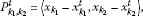(6)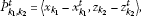(7)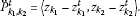(8)

Theorem 2 For the bilinear state space model defined by (1) and (2), we have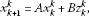(9)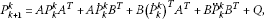(10)

with

$\begin{array}{c}{x}_{k+1}^{k+1}={x}_{k+1}^{k}+{K}_{k+1}\left[{y}_{k}-C{x}_{k+1}^{k}\right],\hfill \\ {P}_{k+1}^{k+1}=\left[I-{K}_{k+1}C\right]{P}_{k+1}^{k},\hfill \\ {\stackrel{˙}{P}}_{k+1}^{k+1}={P}_{k+1}^{k+1}{\left[{V}_{k+1}^{k+1}\right]}^{T},\hfill \\ {\stackrel{¨}{P}}_{k+1}^{k+1}={V}_{k+1}^{k+1}{\stackrel{˙}{P}}_{k+1}^{k+1},\hfill \\ {K}_{k+1}={P}_{k+1}^{k}{C}^{T}{\left[C{P}_{k+1}^{k}{C}^{T}+R\right]}^{-1},\hfill \end{array}$

and

${V}_{k+1}^{k+1}={z}^{\mathrm{\prime }}\left({x}_{k+1}^{k+1}\right)+\frac{1}{2}\mathcal{H}\left({x}_{k+1}^{k},{x}_{k+1}^{k+1}\right),\phantom{\rule{1em}{0ex}}k=0,\dots ,N.$

Proof Equation (9) is obtained by applying the conditional expectation ${E}_{k}\left(\cdot \right)$ to (1):

$\begin{array}{rcl}{x}_{k+1}^{k}& =& {E}_{k}\left({x}_{k+1}\right)\\ =& {E}_{k}\left(A{x}_{k}+B{z}_{k}+{w}_{k}\right)\\ =& A{E}_{k}\left({x}_{k}\right)+B{E}_{k}\left({z}_{k}\right)+{E}_{k}\left({w}_{k}\right)\\ =& A{x}_{k}^{k}+B{z}_{k}^{k}.\end{array}$

To obtain the error recursion (10), we proceed as follows:

$\begin{array}{rcl}{P}_{k+1}^{k}& =& {\parallel {x}_{k+1}-{x}_{k+1}^{k}\parallel }^{2}={\parallel \left(I-{\pi }_{k}\right){x}_{k+1}\parallel }^{2}\\ =& {\parallel \left(I-{\pi }_{k}\right)\left(A{x}_{k}+B{z}_{k}+{w}_{k}\right)\parallel }^{2}\\ =& {\parallel A\left({x}_{k}-{x}_{k}^{k}\right)+B\left({z}_{k}-{z}_{k}^{k}\right)+{w}_{k}\parallel }^{2}\\ =& A{\parallel {x}_{k}-{x}_{k}^{k}\parallel }^{2}{A}^{T}+B{\parallel {z}_{k}-{z}_{k}^{k}\parallel }^{2}{B}^{T}+A〈{x}_{k}-{x}_{k}^{k},{z}_{k}-{z}_{k}^{k}〉{B}^{T}\\ +B〈{z}_{k}-{z}_{k}^{k},{x}_{k}-{x}_{k}^{k}〉{A}^{T}+A〈{x}_{k}-{x}_{k}^{k},{w}_{k}〉+〈{w}_{k},{x}_{k}-{x}_{k}^{k}〉{A}^{T}\\ +B〈{z}_{k}-{z}_{k}^{k},{w}_{k}〉+〈{w}_{k},{z}_{k}-{z}_{k}^{k}〉{B}^{T}+{\parallel {w}_{k}\parallel }^{2}\\ =& A{P}_{k}^{k}{A}^{T}+A{\stackrel{˙}{P}}_{k}^{k}{B}^{T}+B{\left({\stackrel{˙}{P}}_{k}^{k}\right)}^{T}{A}^{T}+B{\stackrel{¨}{P}}_{k}^{k}{B}^{T}+Q.\end{array}$

Now, when $t=k$, we derive the filtering steps. Let

$\begin{array}{rcl}{\rho }_{k}& =& {y}_{k}-{E}_{k-1}\left({y}_{k}\right)=\left(I-{\pi }_{k-1}\right){y}_{k}\\ =& \left(I-{\pi }_{k-1}\right)\left(C{x}_{k}+{v}_{k}\right)={y}_{k}-C{x}_{k}^{k-1}\\ =& C\left({x}_{k}-{x}_{k}^{k-1}\right)+{v}_{k},\phantom{\rule{1em}{0ex}}k=1,\dots ,N.\end{array}$

Then, the mean of the innovations is given by

${E}_{k-1}\left({\rho }_{k}\right)={\pi }_{k-1}\left(I-{\pi }_{k-1}\right){y}_{k}=0,$

and the variance

$\begin{array}{rcl}{\mathrm{\Sigma }}_{k+1}& =& {\parallel {\rho }_{k+1}\parallel }^{2}\\ =& {\parallel C\left({x}_{k+1}-{x}_{k+1}^{k}\right)+{v}_{k+1}\parallel }^{2}\\ =& C{\parallel {x}_{k+1}-{x}_{k+1}^{k}\parallel }^{2}{C}^{T}+{\parallel {v}_{k+1}\parallel }^{2}\\ =& C{P}_{k+1}^{k}{C}^{T}+R.\end{array}$

Also,

$\begin{array}{rcl}〈{\rho }_{k+1},{y}_{k}〉& =& 〈{y}_{k+1}-{y}_{k+1}^{k},{y}_{k}〉\\ =& 〈\left(I-{\pi }_{k}\right){y}_{k+1},{y}_{k}〉=〈{y}_{k+1},\left(I-{\pi }_{k}\right){y}_{k}〉=0,\end{array}$

which means that the innovations are orthogonal to the past measurements. On the other hand,

$\begin{array}{rcl}〈{x}_{k+1},{\rho }_{k+1}〉& =& 〈{x}_{k+1},C\left({x}_{k+1}-{x}_{k+1}^{k}\right)+{v}_{k+1}〉\\ =& 〈{x}_{k+1},{x}_{k+1}-{x}_{k+1}^{k}〉{C}^{T}\\ =& 〈{x}_{k+1},\left(I-{\pi }_{k}\right){x}_{k+1}〉{C}^{T}\\ =& 〈\left(I-{\pi }_{k}\right){x}_{k+1},\left(I-{\pi }_{k}\right){x}_{k+1}〉{C}^{T}\\ =& {\parallel \left(I-{\pi }_{k}\right){x}_{k+1}\parallel }^{2}{C}^{T}\\ =& {P}_{k+1}^{k}{C}^{T}.\end{array}$

From these results, we conclude that ${x}_{k+1}$ and ${\rho }_{k+1}$ have a Gaussian joint distribution conditional on ${Y}_{k}$. That is,

$\left\{\left(\begin{array}{c}{x}_{k+1}\\ {\rho }_{k+1}\end{array}\right)|{\left\{{y}_{t}\right\}}_{1}^{k}\right\}\sim N\left\{\left(\begin{array}{c}{x}_{k+1}^{k}\\ 0\end{array}\right),\left(\begin{array}{cc}{P}_{k+1}^{k}& {P}_{k+1}^{k}{C}^{T}\\ C{P}_{k+1}^{k}& {\mathrm{\Sigma }}_{k+1}\end{array}\right)\right\}.$

Now, since ${Y}_{k}$, ${\rho }_{k+1}$ are orthogonal,

$\begin{array}{rcl}{x}_{k+1}^{k+1}& =& {\pi }_{k+1}\left({x}_{k+1}\right)={\pi }_{\left\{{Y}_{k},{\rho }_{k+1}\right\}}\left({x}_{k+1}\right)\\ =& {\pi }_{{Y}_{k}}\left({x}_{k+1}\right)+{\pi }_{{\rho }_{k+1}}\left({x}_{k+1}\right)\\ =& {E}_{k}\left({x}_{k+1}\right)+〈{x}_{k+1},{\rho }_{k+1}〉{\mathrm{\Sigma }}_{k+1}^{-1}{\rho }_{k+1}\\ =& {x}_{k+1}^{k}+{P}_{k+1}^{k}{C}^{T}{\left[C{P}_{k+1}^{k}{C}^{T}+R\right]}^{-1}{\rho }_{k+1}\\ =& {x}_{k+1}^{k}+{K}_{k+1}\left[{y}_{k+1}-C{x}_{k+1}^{k}\right],\end{array}$

where

${K}_{k+1}={P}_{k+1}^{k}{C}^{T}{\left[C{P}_{k+1}^{k}{C}^{T}+R\right]}^{-1}={P}_{k+1}^{k}{C}^{T}{\mathrm{\Sigma }}_{k+1}^{-1}$

represents the Kalman gain.

Next, we derive the recursion for ${P}_{k+1}^{k+1}$. Since ${x}_{k+1}-{x}_{k+1}^{k}=\left({x}_{k+1}-{x}_{k+1}^{k+1}\right)+{\pi }_{{\rho }_{k+1}}\left({x}_{k+1}-{x}_{k+1}^{k}\right)$ is an orthogonal decomposition,

$\begin{array}{rcl}{P}_{k+1}^{k+1}& =& {\parallel {x}_{k+1}-{x}_{k+1}^{k+1}\parallel }^{2}\\ =& {\parallel {x}_{k+1}-{x}_{k+1}^{k}\parallel }^{2}-{\parallel {\pi }_{{\rho }_{k+1}}\left({x}_{k+1}-{x}_{k+1}^{k}\right)\parallel }^{2}\\ =& {P}_{k+1}^{k}-{\parallel 〈{x}_{k+1}-{x}_{k+1}^{k},{\rho }_{k+1}〉{\mathrm{\Sigma }}_{k+1}^{-1}{\rho }_{k+1}\parallel }^{2}\\ =& {P}_{k+1}^{k}-{\parallel {P}_{k+1}^{k}{C}^{T}{\mathrm{\Sigma }}_{k+1}^{-1}{\rho }_{k+1}\parallel }^{2}\\ =& {P}_{k+1}^{k}-{P}_{k+1}^{k}{C}^{T}{\mathrm{\Sigma }}_{k+1}^{-1}{\parallel {\rho }_{k+1}\parallel }^{2}{\mathrm{\Sigma }}_{k+1}^{-1}C{P}_{k+1}^{k}\\ =& {P}_{k+1}^{k}-{P}_{k+1}^{k}{C}^{T}{\mathrm{\Sigma }}_{k+1}^{-1}C{P}_{k+1}^{k}\\ =& {P}_{k+1}^{k}-{K}_{k+1}C{P}_{k+1}^{k}\\ =& \left[I-{K}_{k+1}C\right]{P}_{k+1}^{k}.\end{array}$

The equation for ${\stackrel{˙}{P}}_{k+1}^{k+1}$ is obtained as follows:

$\begin{array}{rcl}{\stackrel{˙}{P}}_{k+1}^{k+1}& =& 〈{x}_{k+1}-{x}_{k+1}^{k+1},{z}_{k+1}-{z}_{k+1}^{k+1}〉\\ =& 〈{x}_{k+1}-{x}_{k+1}^{k+1},{V}_{k+1}^{k+1}\left({x}_{k+1}-{x}_{k+1}^{k+1}\right)〉\\ =& 〈{x}_{k+1}-{x}_{k+1}^{k+1},{x}_{k+1}-{x}_{k+1}^{k+1}〉{\left[{V}_{k+1}^{k+1}\right]}^{T}\\ =& {P}_{k+1}^{k+1}{\left[{V}_{k+1}^{k+1}\right]}^{T}.\end{array}$

Finally, for ${\stackrel{¨}{P}}_{k+1}^{k+1}$ we have

$\begin{array}{rcl}{\stackrel{¨}{P}}_{k+1}^{k+1}& =& 〈{z}_{k+1}-{z}_{k+1}^{k+1},{z}_{k+1}-{z}_{k+1}^{k+1}〉\\ =& {V}_{k+1}^{k+1}〈{x}_{k+1}-{x}_{k+1}^{k+1},{x}_{k+1}-{x}_{k+1}^{k+1}〉{\left[{V}_{k+1}^{k+1}\right]}^{T}\\ =& {V}_{k+1}^{k+1}{P}_{k+1}^{k+1}{\left[{V}_{k+1}^{k+1}\right]}^{T}\\ =& {V}_{k+1}^{k+1}{\stackrel{˙}{P}}_{k+1}^{k+1}.\end{array}$

This completes the proof. □

We summarize the bilinear Kalman filter as follows: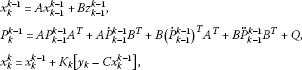(11)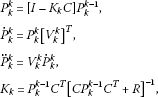(12)

and

${V}_{k}^{k}={z}^{\mathrm{\prime }}\left({x}_{k}^{k}\right)+\frac{1}{2}\mathcal{H}\left({x}_{k}^{k-1},{x}_{k}^{k}\right),\phantom{\rule{1em}{0ex}}k=0,\dots ,N.$

Also, note that the bilinear Kalman filter algorithm is a generalization of the Kalman filter for the linear case which is given in .

### 3.2 A bilinear Kalman smoother

In this subsection, we will develop a Kalman smoother for the bilinear system (1) and (2). We will use the following notation:

$\begin{array}{c}{P}_{{k}_{1},{k}_{2}}^{N}=〈{x}_{{k}_{1}}-{x}_{{k}_{1}}^{N},{x}_{{k}_{2}}-{x}_{{k}_{2}}^{N}〉,\hfill \\ {\stackrel{˙}{P}}_{{k}_{1},{k}_{2}}^{N}=〈{x}_{{k}_{1}}-{x}_{{k}_{1}}^{N},{z}_{{k}_{2}}-{z}_{{k}_{2}}^{N}〉,\hfill \\ {\stackrel{¨}{P}}_{{k}_{1},{k}_{2}}^{N}=〈{z}_{{k}_{1}}-{z}_{{k}_{1}}^{N},{z}_{{k}_{2}}-{z}_{{k}_{2}}^{N}〉.\hfill \end{array}$

Lemma 3 Let

${ϵ}_{k+1}=\left\{{v}_{k+1},\dots ,{v}_{N},{w}_{k+2},\dots ,{w}_{N}\right\}.$
(13)

Then for $1\le k\le N-1$ and with the approximation (5),

$L{\left\{{y}_{m}\right\}}_{1}^{N}=L\left\{{\left\{{y}_{m}\right\}}_{1}^{k},{x}_{k+1}-{x}_{k+1}^{k},{ϵ}_{k+1}\right\},$
(14)

where $L\left\{\cdot \right\}$ denotes the subspace spanned by $\left\{\cdot \right\}$.

Proof Recall that

${z}_{m}={z}_{m}^{N}+{V}_{m}^{N}\left({x}_{m}-{x}_{m}^{N}\right),$

that is,

$\left({z}_{m}-{z}_{m}^{N}\right)\in L\left\{{x}_{m}-{x}_{m}^{N}\right\}.$

Since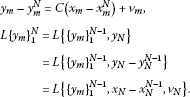Similarly, sinceContinuing in this manner, we get (14). □

We state the bilinear Kalman smoother in the following theorem.

Theorem 4 Consider the bilinear state space model (1) and (2) with ${x}_{N}^{N}$ and ${P}_{N}^{N}$ as given in (11) and (12). Then for $k=N-1,\dots ,1$, we have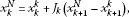(15)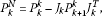(16)

where

${J}_{k}=\left[{P}_{k}^{k}{A}^{T}+{\stackrel{˙}{P}}_{k}^{k}{B}^{T}\right]{\left[{P}_{k+1}^{k}\right]}^{-1}.$

Proof Noting the mutual orthogonality of ${\left\{y\right\}}_{1}^{k}$, $\left\{{x}_{k+1}-{x}_{k+1}^{k}\right\}$ and ${ϵ}_{k+1}$ and the orthogonality of ${x}_{k}$ and ${ϵ}_{k+1}$,

$\begin{array}{rcl}{x}_{k}^{N}& =& {\pi }_{N}{x}_{k}={\pi }_{k}{x}_{k}+{\pi }_{\left({x}_{k+1}-{x}_{k+1}^{k}\right)}{x}_{k}\\ =& {x}_{k}^{k}+〈{x}_{k},{x}_{k+1}-{x}_{k+1}^{k}〉{\parallel {x}_{k+1}-{x}_{k+1}^{k}\parallel }^{-2}\left({x}_{k+1}-{x}_{k+1}^{k}\right)\\ =& {x}_{k}^{k}+〈{x}_{k},{x}_{k+1}-{x}_{k+1}^{k}〉{\left[{P}_{k+1}^{k}\right]}^{-1}\left({x}_{k+1}-{x}_{k+1}^{k}\right).\end{array}$

Now,

$\begin{array}{rcl}〈{x}_{k},{x}_{k+1}-{x}_{k+1}^{k}〉& =& 〈{x}_{k},A{x}_{k}+B{z}_{k}+{w}_{k}-{x}_{k+1}^{k}〉\\ =& 〈{x}_{k},A\left({x}_{k}-{x}_{k}^{k}\right)+B\left({z}_{k}-{z}_{k}^{k}\right)〉\\ =& 〈{x}_{k}-{x}_{k}^{k},{x}_{k}-{x}_{k}^{k}〉{A}^{T}+〈{x}_{k}-{x}_{k}^{k},{z}_{k}-{z}_{k}^{k}〉{B}^{T}\\ =& {P}_{k}^{k}{A}^{T}+{\stackrel{˙}{P}}_{k}^{k}{B}^{T}.\end{array}$

Thus,

$\begin{array}{rcl}{x}_{k}^{N}& =& {x}_{k}^{k}+\left[{P}_{k}^{k}{A}^{T}+{\stackrel{˙}{P}}_{k}^{k}{B}^{T}\right]{\left[{P}_{k+1}^{k}\right]}^{-1}\left({x}_{k+1}-{x}_{k+1}^{k}\right)\\ =& {x}_{k}^{k}+{J}_{k}\left({x}_{k+1}-{x}_{k+1}^{k}\right).\end{array}$

Equation (15) now follows by taking the projection ${\pi }_{N}$ again of both sides and noting that $k\le N$. To derive (16), we compute

$\begin{array}{rcl}{P}_{k}^{N}& =& {\parallel {x}_{k}-{x}_{k}^{N}\parallel }^{2}={\parallel {x}_{k}-{x}_{k}^{k}-{J}_{k}\left({x}_{k+1}-{x}_{k+1}^{k}\right)\parallel }^{2}\\ =& {\parallel {x}_{k}-{x}_{k}^{k}\parallel }^{2}-〈{x}_{k}-{x}_{k}^{k},{x}_{k+1}-{x}_{k+1}^{k}〉{J}_{k}^{T}-{J}_{k}〈{x}_{k+1}-{x}_{k+1}^{k},{x}_{k}-{x}_{k}^{k}〉+{J}_{k}{P}_{k+1}^{k}{J}_{k}^{T}\\ =& {P}_{k}^{k}-〈\left(1-{\pi }_{k}\right){x}_{k},{x}_{k+1}〉{J}_{k}^{T}-{J}_{k}〈{x}_{k+1},\left(1-{\pi }_{k}\right){x}_{k}〉+{J}_{k}{P}_{k+1}^{k}{J}_{k}^{T}\\ =& {P}_{k}^{k}-〈\left(1-{\pi }_{k}\right){x}_{k},A{x}_{k}+B{z}_{k}〉{J}_{k}^{T}-{J}_{k}〈A{x}_{k}+B{z}_{k},\left(1-{\pi }_{k}\right){x}_{k}〉+{J}_{k}{P}_{k+1}^{k}{J}_{k}^{T}\\ =& {P}_{k}^{k}-\left({P}_{k}^{k}{A}^{T}+{\stackrel{˙}{P}}_{k}^{k}{B}^{T}\right){J}_{k}^{T}-{J}_{k}\left(A{P}_{k}^{k}+B{\stackrel{˙}{P}}_{k}^{k}\right)+{J}_{k}{P}_{k+1}^{k}{J}_{k}^{T}\\ =& {P}_{k}^{k}-{J}_{k}{P}_{k+1}^{k}{J}_{k}^{T}-{J}_{k}{P}_{k+1}^{k}{J}_{k}^{T}+{J}_{k}{P}_{k+1}^{k}{J}_{k}^{T}={P}_{k}^{k}-{J}_{k}{P}_{k+1}^{k}{J}_{k}^{T},\end{array}$

which completes the proof. □

The next theorem states the bilinear lag-one recursions.

Theorem 5 Consider the bilinear state space model (1) and (2). Then

$\begin{array}{c}{P}_{k+1,k}^{N}=A{P}_{k}^{N}+B{\left({\stackrel{˙}{P}}_{k}^{N}\right)}^{T},\hfill \\ {\stackrel{˙}{P}}_{k+1,k}^{N}={P}_{k+1,k}^{N}{\left[{V}_{k}^{N}\right]}^{T}.\hfill \end{array}$

Proof Using the definitions in (6) and (7),

$\begin{array}{rcl}{P}_{k+1,k}^{N}& =& 〈{x}_{k+1}-{x}_{k+1}^{N},{x}_{k}-{x}_{k}^{N}〉=〈\left(1-{\pi }_{N}\right){x}_{k+1},\left(1-{\pi }_{N}\right){x}_{k}〉\\ =& 〈{x}_{k+1},\left(1-{\pi }_{N}\right){x}_{k}〉=〈A{x}_{k}+B{z}_{k}+{w}_{k},\left(1-{\pi }_{N}\right){x}_{k}〉\\ =& A〈{x}_{k},\left(1-{\pi }_{N}\right){x}_{k}〉+B〈{z}_{k},\left(1-{\pi }_{N}\right){x}_{k}〉\\ =& A{P}_{k}^{N}+B{\left({\stackrel{˙}{P}}_{k}^{N}\right)}^{T}.\end{array}$

Also,

$\begin{array}{rcl}{\stackrel{˙}{P}}_{k+1,k}^{N}& =& 〈{x}_{k+1}-{x}_{k+1}^{N},{z}_{k}-{z}_{k}^{N}〉\\ =& 〈{x}_{k+1}-{x}_{k+1}^{N},{V}_{k}^{N}\left({x}_{k}-{x}_{k}^{N}\right)〉\\ =& 〈{x}_{k+1}-{x}_{k+1}^{N},{x}_{k}-{x}_{k}^{N}〉{\left[{V}_{k}^{N}\right]}^{T}\\ =& {P}_{k+1,k}^{N}{\left[{V}_{k}^{N}\right]}^{T}.\end{array}$

□

## 4 The bilinear EM algorithm

The unknown parameter set $\theta =\left\{A,B,C,Q,R,V,\mu \right\}$ is estimated by the EM algorithm that iteratively updates the current estimate $\theta \left(i\right)$ of θ by maximizing the log-likelihood function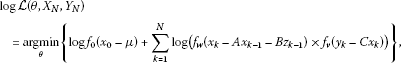(17)

where

• ${f}_{0}\left(\cdot \right)$ represents the n-variate normal density of the initial state ${x}_{0}$ with mean μ and the covariance matrix V.

• ${f}_{v}\left(\cdot \right)$ represents the p-variate normal density with zero mean and the covariance matrix R.

• ${f}_{w}\left(\cdot \right)$ represents the n-variate normal density function with zero mean and the covariance matrix Q.

The conditional expectation step (E-step) finds the missing data, i.e., ${X}_{N}$, given the observed data and current estimated parameters, and then substitutes these expectations for the missing data. Specifically, let $\theta \left(i-1\right)$ be the current estimate of the parameter θ, then the E-step finds the conditional expectation $\mathrm{E}\left\{\cdot \right\}$ of the complete-data log-likelihood given $\theta \left(i-1\right)$:

$q\left(\theta |\theta \left(i-1\right)\right)=\mathrm{E}\left\{log\mathcal{L}\left(\theta ,{X}_{N},{Y}_{N}\right)|{Y}_{N},\theta \left(i-1\right)\right\}.$
(18)

The M-step determines $\theta \left(i\right)$ by maximizing the expected complete-data log-likelihood

$q\left(\theta \left(i\right)|\theta \left(i-1\right)\right)\ge q\left(\theta |\theta \left(i-1\right)\right),\phantom{\rule{1em}{0ex}}\mathrm{\forall }\theta .$

The following theorem accomplishes the expectation step.

Theorem 6 For the bilinear state space model (1) and (2),

$\begin{array}{rcl}q\left(\theta \left(i\right)|\theta \left(i-1\right)\right)& =& -\frac{1}{2}log|V|-\frac{1}{2}Tr\left\{{V}^{-1}\left(\mathrm{\Delta }-{\stackrel{ˆ}{x}}_{0}{\mu }^{T}-\mu {\stackrel{ˆ}{x}}_{0}^{T}+\mu {\mu }^{T}\right)\right\}\\ -\frac{N}{2}log|Q|-\frac{1}{2}Tr\left\{{Q}^{-1}\left(\mathrm{\Theta }-\mathrm{\Psi }{A}^{T}-\mathrm{\Pi }{B}^{T}-A{\mathrm{\Psi }}^{T}\\ +A\mathrm{\Phi }{A}^{T}-B{\mathrm{\Pi }}^{T}+B\mathrm{\Lambda }{B}^{T}\right)\right\}\\ -\frac{N}{2}log|R|-\frac{1}{2}Tr\left\{{R}^{-1}\left(\delta -\mathrm{\Omega }{C}^{T}-C{\mathrm{\Omega }}^{T}+C\mathrm{\Phi }{C}^{T}\right)\right\}+\mathrm{const},\end{array}$

where

$\begin{array}{c}\mathrm{\Delta }={E}_{N}\left({x}_{0}{x}_{0}^{T}\right),\phantom{\rule{2em}{0ex}}{\stackrel{ˆ}{x}}_{0}={E}_{N}\left({x}_{0}\right),\hfill \\ \mathrm{\Theta }=\sum _{k=1}^{N}\left({x}_{k}^{N}{\left({x}_{k}^{N}\right)}^{T}+{P}_{k}^{N}\right),\phantom{\rule{2em}{0ex}}\mathrm{\Psi }=\sum _{k=1}^{N}\left({x}_{k}^{N}{\left({x}_{k-1}^{N}\right)}^{T}+{P}_{k,k-1}^{N}\right),\hfill \\ \mathrm{\Pi }=\sum _{k=1}^{N}\left({x}_{k}^{N}{\left({z}_{k-1}^{N}\right)}^{T}+{\stackrel{˙}{P}}_{k,k-1}^{N}\right),\phantom{\rule{2em}{0ex}}\mathrm{\Phi }=\sum _{k=1}^{N}\left({x}_{k-1}^{N}{\left({x}_{k-1}^{N}\right)}^{T}+{P}_{k-1}^{N}\right),\hfill \\ \mathrm{\Gamma }=\sum _{k=1}^{N}{E}_{N}\left({x}_{k-1}{z}_{k-1}^{T}\right),\phantom{\rule{2em}{0ex}}\mathrm{\Lambda }=\sum _{k=0}^{N-1}\left({z}_{k}^{N}{\left({z}_{k}^{N}\right)}^{T}+{\stackrel{¨}{P}}_{k}^{N}\right),\hfill \\ \mathrm{\Omega }=\sum _{k=1}^{N}{x}_{k}^{N}{y}_{k}^{T},\phantom{\rule{2em}{0ex}}\delta =\sum _{k=1}^{N}{\parallel {y}_{k}\parallel }^{2}.\hfill \end{array}$

Proof Since the system is Markovian, we may use Bayes’ rule successively to get

$p\left(\theta ,{X}_{N},{Y}_{N}\right)=p\left({y}_{1},\dots ,{y}_{N},{x}_{0},\dots ,{x}_{N}\right)$
(19)
$=p\left({x}_{0}\right)\prod _{k=1}^{N}p\left({y}_{k}|{x}_{k}\right)\prod _{k=0}^{N-1}p\left({x}_{k+1}|{x}_{k}\right).$
(20)

From the assumptions on ${x}_{0}$, ${w}_{k}$, and ${v}_{k}$, the density functions $p\left({x}_{0}\right)$, $p\left({y}_{k}|{x}_{k}\right)$, and $p\left({x}_{k+1}|{x}_{k}\right)$ are given by

$\begin{array}{c}p\left({x}_{0}\right)=\frac{1}{{\left(2\pi \right)}^{\frac{n}{2}}|V{|}^{\frac{1}{2}}}exp\left\{-\frac{1}{2}{\left({x}_{0}-\mu \right)}^{T}{V}^{-1}\left({x}_{0}-\mu \right)\right\},\hfill \\ p\left({x}_{k+1}|{x}_{k}\right)=\frac{1}{{\left(2\pi \right)}^{\frac{n}{2}}|Q{|}^{\frac{1}{2}}}exp\left\{-\frac{1}{2}{\left({x}_{k+1}-A{x}_{k}-B{z}_{k}\right)}^{T}{Q}^{-1}\left({x}_{k+1}-A{x}_{k}-B{z}_{k}\right)\right\},\hfill \end{array}$

and

$p\left({y}_{k}|{x}_{k}\right)=\frac{1}{{\left(2\pi \right)}^{\frac{n}{2}}|R{|}^{\frac{1}{2}}}exp\left\{-\frac{1}{2}{\left({y}_{k}-C{x}_{k}\right)}^{T}{R}^{-1}\left({y}_{k}-C{x}_{k}\right)\right\}.$

Now, substituting these densities in (17) and taking the logarithm of both sides, we get

$\begin{array}{rcl}\mathcal{L}\left(\theta ,{X}_{N},{Y}_{N}\right)& =& -\frac{1}{2}log|V|-\frac{1}{2}{\left({x}_{0}-\mu \right)}^{T}{V}^{-1}\left({x}_{0}-\mu \right)\\ -\frac{N}{2}log|Q|-\frac{1}{2}\sum _{k=0}^{N-1}{\left({x}_{k+1}-A{x}_{k}-B{z}_{k}\right)}^{T}{Q}^{-1}\left({x}_{k+1}-A{x}_{k}-B{z}_{k}\right)\\ -\frac{N}{2}log|R|-\frac{1}{2}\sum _{k=1}^{N}{\left({y}_{k}-C{x}_{k}\right)}^{T}{R}^{-1}\left({y}_{k}-C{x}_{k}\right)+\mathrm{const}.\end{array}$

The result follows upon taking the expectation conditional on ${Y}_{N}$, making use of

$\begin{array}{c}{E}_{N}\left({x}^{T}Ay\right)=Tr\left[A{E}_{N}\left(x{y}^{T}\right)\right],\hfill \\ {E}_{N}\left({x}_{k}{z}_{k}^{T}\right)=0,\hfill \\ {E}_{N}\left({x}_{k+1}{z}_{k}^{T}\right)=B{E}_{N}\left({z}_{k}{z}_{k}^{T}\right),\hfill \end{array}$

and simplifying. The middle equality follows from the fact that odd moments of Gaussian random variables vanish. □

The computation of Θ, Φ, Ψ, Π and Λ given a current estimate $\theta \left(i\right)$ of θ involves the use of the bilinear Kalman filter and smoother introduced in Sections 3.1 and 3.2. For this purpose, we introduce

$\begin{array}{c}\mathrm{\Theta }=\sum _{k=1}^{N}{E}_{t}\left({x}_{k}{x}_{k}^{T}\right)=\sum _{k=1}^{N}\left({x}_{k}^{t}{\left({x}_{k}^{t}\right)}^{T}+{P}_{k}^{t}\right),\hfill \\ \mathrm{\Phi }=\sum _{k=1}^{N}\left({x}_{k-1}^{t}{\left({x}_{k-1}^{t}\right)}^{T}+{P}_{k-1}^{t}\right),\phantom{\rule{2em}{0ex}}\mathrm{\Psi }=\sum _{k=1}^{N}\left({x}_{k}^{t}{\left({x}_{k-1}^{t}\right)}^{T}+{P}_{k,k-1}^{t}\right),\hfill \\ \mathrm{\Pi }=\sum _{k=1}^{N}\left({x}_{k}^{t}{\left({z}_{k-1}^{t}\right)}^{T}+{\stackrel{˙}{P}}_{k,k-1}^{t}\right),\phantom{\rule{2em}{0ex}}\mathrm{\Lambda }=\sum _{k=0}^{N-1}\left({z}_{k}^{t}{\left({z}_{k}^{t}\right)}^{T}+{\stackrel{¨}{P}}_{k}^{t}\right).\hfill \end{array}$

The next step of the EM algorithm is to maximize the function $q\left(\theta \left(i\right)|\theta \left(i-1\right)\right)$ with respect to θ.

Theorem 7 The maximizer of $q\left(\theta \left(i\right)|\theta \left(i-1\right)\right)$ is obtained for the parameter vector θ given by(21)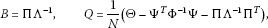(22)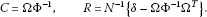(23)

Proof Let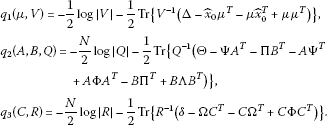Then

$q\left(\theta \left(i\right)|\theta \left(i-1\right)\right)={q}_{1}\left(\mu ,V\right)+{q}_{2}\left(A,B,Q\right)+{q}_{3}\left(C,R\right)+\mathrm{const},$

which means that $q\left(\theta \left(i\right),\theta \left(i-1\right)\right)$ is maximized by separately minimizing ${q}_{1}$, ${q}_{2}$, ${q}_{3}$. This is done by setting the partial derivative of q with respect to each parameter equal to zero (i.e., $\frac{\partial q}{\partial x}=0$) and solving the resulting system of equations. □

The EM algorithm for a bilinear state space model is summarized as follows.

Bilinear EM algorithm

1. 1.

Initialize the EM algorithm by choosing initial values of $\theta \left(0\right)$.

2. 2.

Calculate the incomplete-data likelihood, $logL\left({Y}_{n};\theta \right)$.

3. 3.

Execute the E-step by using the bilinear Kalman filter and smoother in (9)-(10) and (15)-(16), respectively.

4. 4.

Execute the M-step using (21)-(23) and update the estimates of θ using (M-step) to obtain $\theta \left(i\right)$.

5. 5.

Repeat Steps 2 to 4 until convergence.

## 5 Simulation results

A $1\text{,}000$ Monte Carlo simulation is performed to illustrate the utility of the bilinear algorithm. The observed data are generated according to the second-order bilinear state space model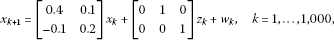(24)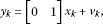(25)

where ${w}_{k}$ and ${v}_{k}$ are independent identically distributed (i.i.d.) Gaussian noises such that

$\begin{array}{c}{w}_{k}\sim \mathrm{N}\left(0,0.01×{I}_{2}\right),\hfill \\ {v}_{k}\sim \mathrm{N}\left(0,0.01\right).\hfill \end{array}$

In all simulations, the number of iterations for the EM algorithm is fixed and its value set to $J=100$.

Figure 1 shows a sample of realizations of the input noise ${w}_{k}$, and Figure 2 shows the output noise ${v}_{k}$, respectively. Figure 3 compares the observed output signals and the estimated output signal. The average estimates of the parameters are

$\begin{array}{c}A=\left[\begin{array}{cc}0.3891& 0.1015\\ -0.09812& 0.2117\end{array}\right],\phantom{\rule{2em}{0ex}}B=\left[\begin{array}{ccc}0.0012& 1.1016& 0\\ 0& -0.0345& 0.9761\end{array}\right],\hfill \\ C=\left[\begin{array}{cc}-0.0927& 1.014\end{array}\right].\hfill \end{array}$

The mean square error (MSE) is defined as

${E}_{N}=\frac{1}{N}\sum _{k=1}^{N}{\left({y}_{k}-C{x}_{k|k-1}\right)}^{2},$

and its value for $1\text{,}000$ run for different values of $cov\left(R\right)$ and $cov\left(Q\right)$ is kept constant, which is shown in Table 1.

## 6 Application to wind speed

In this section, we apply the proposed bilinear algorithm to the daily averaged wind speed data for Arar, a city located in the north eastern region of the kingdom of Saudi Arabia for a period of $16\frac{1}{2}$ years as shown in Figure 4. It should be noted that all the calculations are carried out on normalized time series data.

To estimate the dimension of the state in the state space model, we apply the stochastic subspace system identification algorithm described in . This is done by constructing the singular value diagram of the block Hankel matrix for the normalized wind speed data as shown in Figure 5. That is, the dimension of the state equals the number of the significant singular values; here $n=2$. For clarity, we compare the observed wind speed values with the estimated ones using a linear model  with our proposed algorithm for a period of 100 days as shown in Figure 6. The estimated parameters for the linear state space model are

$A=\left[\begin{array}{cc}0.9475& 0.1991\\ 0.1968& 0.0935\end{array}\right],\phantom{\rule{2em}{0ex}}C=\left[\begin{array}{cc}0.5355& 0.3492\end{array}\right],$

and for the bilinear state space model, they are

$\begin{array}{c}A=\left[\begin{array}{cc}0.9475& 0.1991\\ 0.1968& 0.0935\end{array}\right],\phantom{\rule{2em}{0ex}}C=\left[\begin{array}{cc}0.5355& 0.3492\end{array}\right],\hfill \\ B=\left[\begin{array}{ccc}0& 1.092& -1.092\\ 0.01092& 0.0557& 0.1092\end{array}\right].\hfill \end{array}$

The MSE for the estimated wind speed data using the linear EM algorithm is 0.3864, and 0.0197 for the bilinear EM algorithm.

## References

1. Krener AJ: Bilinear and nonlinear realizations of input-output maps. SIAM J. Control 1975, 13(4):827–834. 10.1137/0313049

2. Pardalos PM, Yatsenko V: Optimization and Control of Bilinear Systems: Theory, Algorithms and Applications. Springer, Berlin; 2008.

3. Shumway R, Stoffer D: An approach to time series smoothing and forecasting using the EM algorithm. J. Time Ser. Anal. 1982, 3(4):253–264. 10.1111/j.1467-9892.1982.tb00349.x

4. Strgate SH: Nonlinear Dynamics and Chaos, with Applications to Physics, Biology, Chemistry, and Engineering. Perseus Books, New York; 1994.

5. Galanis G, Anadranistakis M: A one-dimension Kalman filter for correction of near surface temperature forecasts. Meteorol. Appl. 2002, 9: 437–441. 10.1017/S1350482702004061

6. Goel NS: On the Volterra and Other Nonlinear Models of Interacting Populations. Academic Press, San Diego; 1971.

7. Lorenz EN, Emanuel KE: Optimal sites for supplementary weather observations: simulations with a small model. J. Atmos. Sci. 1998, 55: 399–414. 10.1175/1520-0469(1998)055<0399:OSFSWO>2.0.CO;2

8. Lorenz EN: Designing chaotic models. J. Atmos. Sci. 2005, 62: 1574–1588. 10.1175/JAS3430.1

9. Monbet V, Ailliot P, Prevosto M: Survey of stochastic models for wind and sea state time series. Probab. Eng. Mech. 2007, 22: 113–126. 10.1016/j.probengmech.2006.08.003

10. Roy D, Musielak ZE: Generalized Lorenz models and their routes to chaos. III. Energy-conserving horizontal and vertical mode truncations. Chaos Solitons Fractals 2007, 33: 1064–1070. 10.1016/j.chaos.2006.05.084

11. Preistley MB: Non-Linear and Non-Stationary Time Series Analysis. Academic Press, San Diego; 1989.

12. Gibson S, Wills A, Ninness B: Maximum-likelihood parameter estimation of bilinear systems. IEEE Trans. Autom. Control 2005, 50: 1581–1596.

13. Anderson JL, Anderson SL: A Monte Carlo implementation of the nonlinear filtering problem to produce ensemble assimilations and forecasts. Mon. Weather Rev. 1999, 127: 2741–2758. 10.1175/1520-0493(1999)127<2741:AMCIOT>2.0.CO;2

14. Bendat J: Nonlinear System Analysis and Identification from Random Data. Wiley-Interscience, New York; 1990.

15. Daum FE: Exact finite dimensional nonlinear filters. IEEE Trans. Autom. Control 1986, 31(7):616–622. 10.1109/TAC.1986.1104344

16. Ha QP, Trinh H: State and input simultaneous estimation for a class of nonlinear system. Automatica 2004, 40: 1779–1785. 10.1016/j.automatica.2004.05.012

17. Kailath T, Sayed A, Hassibi B: Linear Estimation. Prentice Hall, New York; 2000.

18. Kerschen G, Worden K, Vakakis AF, Golinval J: Past, present and future of nonlinear system identification in structural dynamics. Mech. Syst. Signal Process. 2006, 20: 505–592. 10.1016/j.ymssp.2005.04.008

19. Ljung L: System Identification: Theory for the User. 2nd edition. Prentice Hall, Upper Saddle River; 1999.

20. Norgaard M, Poulsen NK, Ravn O: New developments in state estimation for nonlinear systems. Automatica 2000, 36: 1627–1638. 10.1016/S0005-1098(00)00089-3

21. Wiener N: Nonlinear Problems in Random Theory. MIT Press, Boston; 1958.

22. Dempster A, Laird N, Rubin D: Maximum likelihood from incomplete data via the EM algorithm. J. R. Stat. Soc. B 1977, 39: 1–38.

23. Tanaka H, Katayama T: A stochastic realization algorithm via block LQ decomposition in Hilbert space. Automatica 2006, 42: 741–746. 10.1016/j.automatica.2005.12.025

## Acknowledgements

The first author was supported by Tayyebah University. The second and third authors would like to thank King Fahd University for the excellent research facilities they provide.

## Author information

Authors

### Corresponding author

Correspondence to A Al-Mazrooei.

### Competing interests

The authors declare that they have no competing interests.

### Authors’ contributions

The authors have achieved equal contributions to each part of this paper. All authors read and approved the final version of the manuscript.

## Authors’ original submitted files for images

Below are the links to the authors’ original submitted files for images.

## Rights and permissions

Open Access This article is distributed under the terms of the Creative Commons Attribution 2.0 International License (https://creativecommons.org/licenses/by/2.0), which permits unrestricted use, distribution, and reproduction in any medium, provided the original work is properly cited.

Reprints and Permissions

Al-Mazrooei, A., Al-Mutawa, J., El-Gebeily, M. et al. Filtering and identification of a state space model with linear and bilinear interactions between the states. Adv Differ Equ 2012, 176 (2012). https://doi.org/10.1186/1687-1847-2012-176

• Accepted:

• Published:

• DOI: https://doi.org/10.1186/1687-1847-2012-176

### Keywords

• bilinear state space model
• Kalman filter and smoother
• maximum likelihood estimate
• EM algorithm